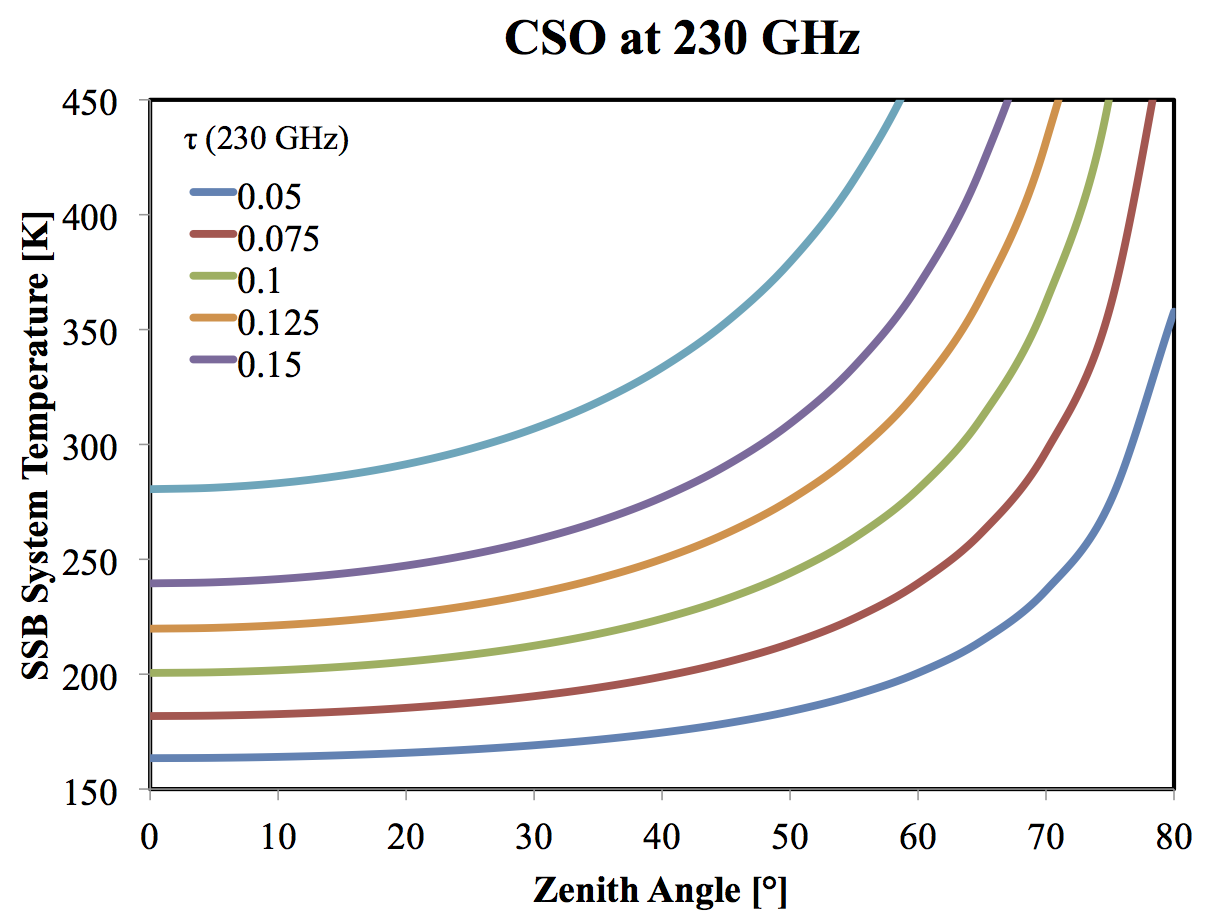# Observing Time

For heterodyne observations, estimate the sensitivity with the radiometer equation:

• Trms = K Tsys (t ∆f )-1/2,

where Tsys is the system temperature, t is the integration time, ∆f is the channel width, and K ≈ 2, depending on the switching scheme and observing strategy. Invert this equation to estimate the necessary observing time. Do not neglect to add overhead for setup, pointing, calibration, etc.

## System Temperature

Estimate system temperature on the TA* scale with:

• Tsys [SSB, TA*] = [exp(τA ) / α] × 2 × (Trx [DSB] + α [1 - exp(-τA )] Tatm + (1 - α ) Tspill ) ,

where

• τ is the atmospheric optical depth at the observing frequency, see model atmospheric spectra,
• A is the airmass = secant (zenith angle),
• α is the hot spillover efficiency = 1 - (fraction of power falling on ground, etc.), ≈ 93%,
• Tatm is the atmospheric temperature, ≈ 280 K,
• Tspill is the warm spillover temperature, ≈ 280 K, and
• the Cosmic (Microwave) Background Radiation has been ignored.

For the above parameters and TRx = 40 K [DSB], the approximate SSB system temperature at 230 GHz is:If you're observing small sources, further adjust for the main beam efficiency.

If the atmospheric optical depth in the two sidebands is different, please review the discussion of sideband correction.